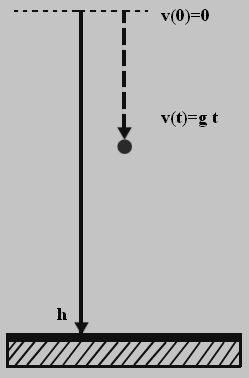# Free Fall Formula

Freefall as the term says, is a body falling freely because of the gravitational pull of our earth.

Imagine a body with velocity (v) is falling freely from a height (h) for time (t) seconds because of gravity (g).Free Fall Formulas are articulated as follows:

h = (1/2) gt2

v2 = 2gh

v = gt

Free fall is independent of the mass of the body. It depends only on the height and the duration the body is flung for.

## Freefall Related Solved Examples

Underneath are given questions on free fall which may be useful for you.

Problem 1: Calculate the body height if it has mass of kg and after 7 seconds it reaches the ground?

Given: Height h =?
Time t = 7s
We all are acquainted with the fact that free fall is independent of mass.

Hence, it is given as

$$\begin{array}{l}h = \frac{1}{2}gt^{2}\end{array}$$

h = 0.5 × 9.8 × (7)2

h = 240.1 m

Problem  2: The cotton falls after 3 s and iron falls after 5 s. Which is moving with higher velocity?